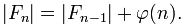# Project Euler – Problem 72 Solution

Check out my new course Learn you some Lambda best practice for great good! and learn the best practices for performance, cost, security, resilience, observability and scalability.

#### Problem

Consider the fraction, n/d, where n and d are positive integers. If n<d and HCF(n,d)=1, it is called a reduced proper fraction.

If we list the set of reduced proper fractions for d <= 8 in ascending order of size, we get:

1/8, 1/7, 1/6, 1/5, 1/4, 2/7, 1/3, 3/8, 2/5, 3/7, 1/2, 4/7, 3/5, 5/8, 2/3, 5/7, 3/4, 4/5, 5/6, 6/7, 7/8

It can be seen that there are 21 elements in this set.

How many elements would be contained in the set of reduced proper fractions for d <= 1,000,000?

#### Solution

```let hasDivisor(n) =
let upperBound = bigint(sqrt(double(n)))
[2I..upperBound] |> Seq.exists (fun x -> n % x = 0I)

let isPrime(n) = if n = 1I then false else not(hasDivisor(n))

// define the sequence of prime numbers
let primeSeq =
Seq.unfold (fun state -> if state >= 3I then Some(state, state+2I) else Some(state, state+1I)) 1I
|> Seq.filter isPrime |> Seq.cache

// define function to find the prime denominators for a number n
let getPrimeFactors n =
let rec getPrimeFactorsRec denominators n =
if n = 1I then denominators
else
let denominator = primeSeq |> Seq.filter (fun x -> n % x = 0I) |> Seq.head
getPrimeFactorsRec (denominators @ [denominator]) (n/denominator)
getPrimeFactorsRec [] n

let totient n =
let primeFactors = getPrimeFactors n |> Seq.distinct
n * (primeFactors |> Seq.map (fun n' -> n'-1I) |> Seq.reduce (*)) / (primeFactors |> Seq.reduce (*))

let f n = [2I..n] |> List.map totient |> List.sum
```

This is almost identical to the solution to problem 69, with the exception of the last two steps. According to the information on Farey sequence on wikipedia:

The Farey sequence of order n contains all of the members of the Farey sequences of lower orders. In particular Fn contains all of the members of Fn?1, and also contains an additional fraction for each number that is less than n and coprime to n. Thus F6 consists of F5 together with the fractions 1?6 and 5?6. The middle term of a Farey sequence Fn is always 1?2, for n > 1.

From this, we can relate the lengths of Fn and Fn?1 using Euler’s totient function ?(n) :Given that F1 = 0 in our case, F2 = 0 + totient 2, F3 = totient 2 + totient 3, and so on, and therefore Fn = totient 2 + totient 3 + … totient n.Hi, I’m Yan. I’m an AWS Serverless Hero and the author of Production-Ready Serverless.

I specialise in rapidly transitioning teams to serverless and building production-ready services on AWS.

Are you struggling with serverless or need guidance on best practices? Do you want someone to review your architecture and help you avoid costly mistakes down the line? Whatever the case, I’m here to help.Check out my new course, Learn you some Lambda best practice for great good! In this course, you will learn best practices for working with AWS Lambda in terms of performance, cost, security, scalability, resilience and observability. Enrol now and enjoy a special preorder price of £9.99 (~\$13).Are you working with Serverless and looking for expert training to level-up your skills? Or are you looking for a solid foundation to start from? Look no further, register for my Production-Ready Serverless workshop to learn how to build production-grade Serverless applications!